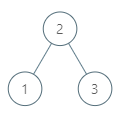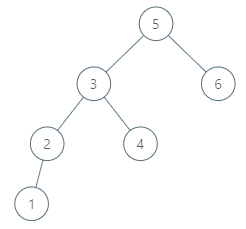## 285. Inorder Successor in BST

Given a binary search tree and a node in it, find the in-order successor of that node in the BST.

The successor of a node `p` is the node with the smallest key greater than `p.val`.

Example 1:```Input: root = [2,1,3], p = 1
Output: 2
Explanation: 1's in-order successor node is 2. Note that both p and the return value is of TreeNode type.
```

Example 2:```Input: root = [5,3,6,2,4,null,null,1], p = 6
Output: null
Explanation: There is no in-order successor of the current node, so the answer is `null`.
```

Note:

1. If the given node has no in-order successor in the tree, return `null`.
2. It's guaranteed that the values of the tree are unique.

## Rust Solution

``````struct Solution;
use rustgym_util::*;

trait Inorder {
fn inorder(&self, p: i32, successor: &mut TreeLink);
}

fn inorder(&self, p: i32, successor: &mut TreeLink) {
if let Some(node) = self {
let node = node.borrow();
node.left.inorder(p, successor);
if successor.is_none() && node.val > p {
*successor = tree!(node.val);
}
node.right.inorder(p, successor);
}
}
}

impl Solution {
let p = p.as_ref().unwrap().borrow().val;
let mut res = None;
root.inorder(p, &mut res);
res
}
}

#[test]
fn test() {
let root = tree!(2, tree!(1), tree!(3));
let p = tree!(1);
let res = tree!(2);
assert_eq!(Solution::inorder_successor(root, p), res);
let root = tree!(5, tree!(3, tree!(2, tree!(1), None), tree!(4)), tree!(6));
let p = tree!(6);
let res = None;
assert_eq!(Solution::inorder_successor(root, p), res);
}
``````

Having problems with this solution? Click here to submit an issue on github.